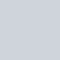# 先來看看今天的結果圖## -> 此篇文章的程式碼 github

Day12_畫線畫矩形畫橢圓_lines_rectangle_ellipse.ipynb

# 運用 OpenCV 畫線、畫矩形、畫橢圓

## 運用 OpenCV 畫線

``````def draw_lines(img):
start_point = (20, 20)
end_point = (120, 120)
color = (0, 255, 0) # green
thickness = 3 # 寬度
cv2.line(img, start_point, end_point, color, thickness)

return img
``````

cv2.line(img, start_point, end_point, color, thickness)

• img 原圖片
• start_point 線條起點
• end_point 線條終點
• color 線條顏色
• thickness 線條粗細## 運用 OpenCV 畫矩形

``````def draw_rectangle(img):
left_up = (20, 60)
right_down =  (180, 120)
color = (0, 0, 255) # red
thickness = 2 # 寬度 (-1 表示填滿)
cv2.rectangle(img, left_up, right_down, color, thickness)

cv2.rectangle(img, (125, 20), (175, 40), (255, 0, 255), -1)

return img
``````

cv2.rectangle(img, left_up, right_down, color, thickness)

• img 原圖片
• left_up 矩形左上角座標
• right_down 矩形右下角座標
• color 矩形顏色
• thickness 矩形線條粗細 (-1 表示填滿)## 運用 OpenCV 畫橢圓

``````def draw_ellipse(img):
center = (100, 100)  #圓心
axes =  (25, 55) #橢圓的長半軸與短半軸的大小
rotateAngle = 45 #橢圓的旋轉角度 (傾斜 +45度)
startAngle = 0 #橢圓弧的起始角度
endAngle = 360 #橢圓弧的終止角度 (180 表示只畫一半)
color = (255, 0, 0) # blue
thickness = 2 # 寬度 (-1 表示填滿)

cv2.ellipse(img, center, axes, rotateAngle, startAngle, endAngle, color, thickness)

cv2.ellipse(img, (50, 50), (20, 30), -45, 0, 180, (0, 255, 255), -1)

return img
``````

cv2.ellipse(img, center, axes, rotateAngle, startAngle, endAngle, color, thickness)

• img 原圖片
• center 圓心
• axes 橢圓的長半軸與短半軸的大小
• rotateAngle 橢圓的旋轉角度 (傾斜角度)
• startAngle 橢圓弧的起始角度
• endAngle 橢圓弧的終止角度 (180 表示只畫一半)
• color 橢圓的顏色
• thickness 橢圓的線條粗細 (-1 表示填滿)【沒錢ps,我用OpenCV!】Day 12 – 基本修圖4，運用 OpenCV 畫線、畫矩形、畫橢圓 draw lines, draw rectangle, draw ellipse

# Reference

https://docs.scipy.org/doc/numpy-1.13.0/reference/generated/numpy.full.html

Python 與 OpenCV 基本讀取、顯示與儲存圖片教學

https://blog.csdn.net/u011520181/article/details/83933325

Python 與 OpenCV 加入線條圖案與文字教學

https://blog.gtwang.org/tag/opencv/

https://www.geeksforgeeks.org/python-opencv-cv2-circle-method/### 1 則留言

1. #### 【PyQt5】Day 17 / Project 製作標註 roi 工具, 開始導入 OpenCV 作為繪圖引擎, 在圖上畫點並顯示座標 - 嗡嗡的隨手筆記

[…] 【OpenCV】12 – 運用 OpenCV 畫線、畫矩形、畫框、畫橢圓 draw lines, draw rectangle,… […]